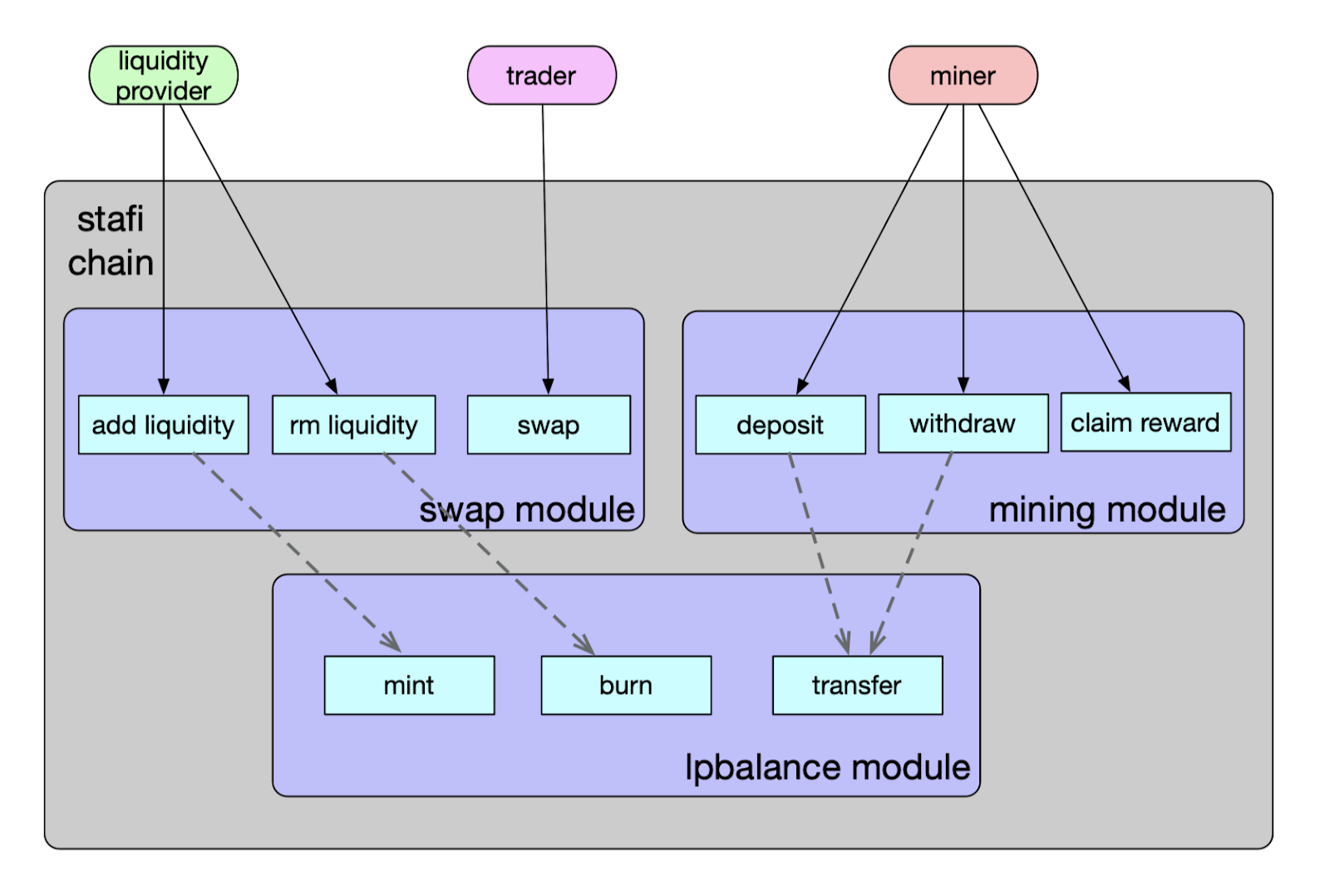# rDEX Architecture

## Target

Provide liquidity for rToken and maintain the value of rToken.
1.Completely decentralized and independent
2.Support to provide liquidity asymmetrically, but encourage to provide liquidity symmetrically
3.Make up for LP’s impermanent losses through shared trading fees and mining incentives

## Architecture

The overall structure is shown in the following figure:When the user swaps tokens on rDEX, the trading fee based on slippage will be charged. If the slippage is too high, the corresponding trading fee will also increase hugely, limiting some large transactions and also compensating the liquidity provider.

## Mining Module

The module mainly provides mining program incentives for Liquidity Provider:
1.Deposit Function: Liquidity provider stakes the LP token to participate in mining program
2.Withdraw Function: The user withdraws LP token and stops mining, and the corresponding mining reward will be issued at this time
3.Claim Function: Withdraw the mining rewards

## Lpbalance Module

This module deals with the functions like minting, burning and transferring LP tokens, etc.

## Formula Derivation

Product formula in Uniswap:
`X*Y=K`
When the number of inputs is `x` and the number of outputs is `y`:
`(X+x)*(Y-y)=K`
`X*Y-X*y+x*Y-x*y=X*Y`
`X*y+x*y=x*Y`
`y=(x*Y)/(X+x)`
If the exchange is made at the exchange price, the output is `y'`:
`y'/Y = x/X`
`y'=(x*Y)/X`
We can further get slippage slip:
`slip=(y'-y)/y'=1-y/y'=1-X/(X+x)=x/(x+X)`
We can see that when `x` is closer to `X`, the slippage is greater.
The trading fee rate in Uniswap is fixed at 0.3%, and rDEX will use a slippage based fee model instead.
If we take slip as the transaction fee rate here:
`fee=slip*y=(x/(x+X))*(x*Y/(X+x))=(x^2*Y)/(X+x)^2`
The output `y''` in the hands of the end user after deducting the handling fee:
`y'=y-fee=(x*Y)/(X+x)-(x^2*Y)/(X+x)^2=(x*X*Y)/(X+x)^2`
Current price: `y1=(x*X1*Y1)/(X1+x)^2`
Pool after exchange: `X2=X1+xY2=Y1-y1`
Price after exchange: `y2=(x*X2*Y2)/(X2+x)^2`

## Formula summary

Calculation formulas for transaction fee, exchange amount, etc.:
`Swap Fee=(x^2*Y)/(x+X)^2`
`Swap Result=(x*X*Y)/(x+X)^2`
`x`=Sent Asset Amount
`X`=Sent Asset Pool Balance
`Y`=Received Asset Pool Balance
Liquidity provider liquidity pool share calculation formula:
`slipAdjustment=(1-ABS((F*r-f*R)/((f+F)(r+R))))`
`units=((P*(r*F+R*f))/(2*R*F))*slipAdjustment`
`f`=Native amount added
`r`=rToken amount added
`F`=Native Balance (before)
`R`=rToken Balance (before)
`P`=existing Pool Units

## rDEX-bot Service

It is an independent service whose main responsibility is to maintain the balance between the rToken trading price on rDEX and the rToken exchange rate on the StaFi chain.